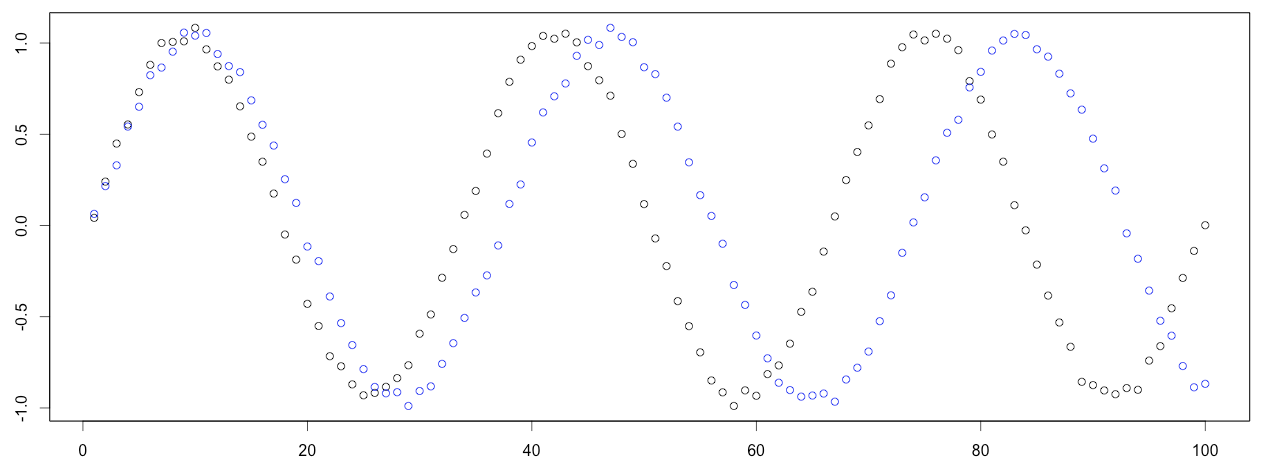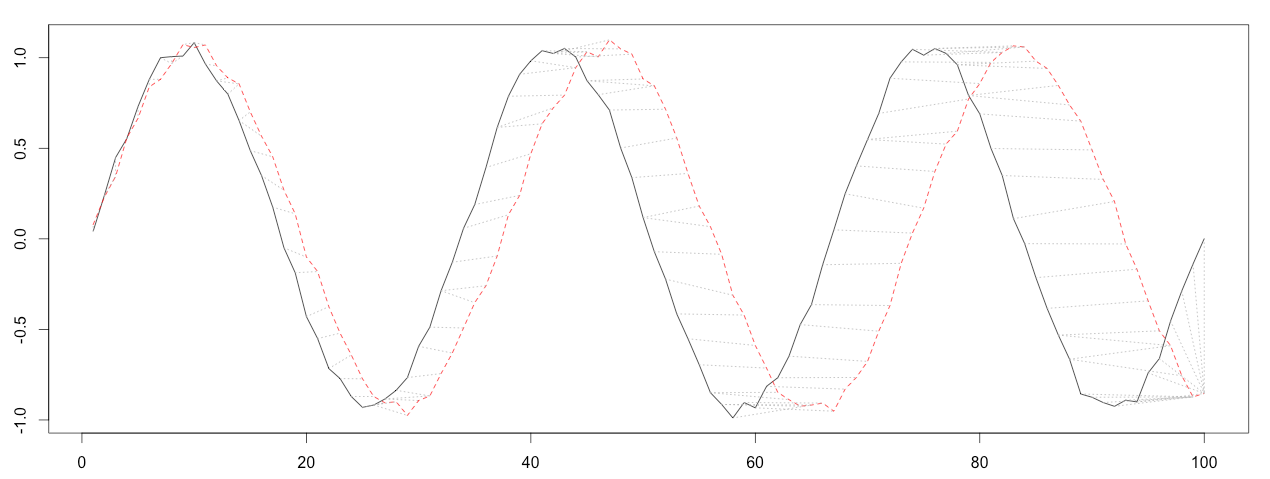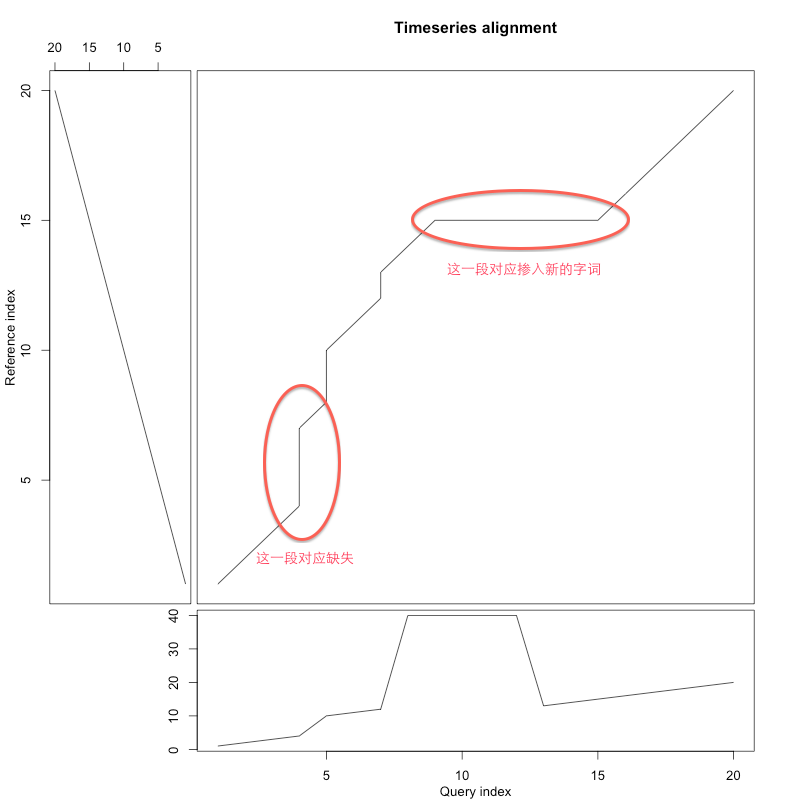# 算法笔记-DTW动态时间规整

## 1.简介DTW能够计算这两个序列的相似程度，并且给出一个能最大程度降低两个序列距离的点到点的匹配。见下图，其中黑色与红色曲线中的虚线就是表示点点之间的一个对应关系。## 2.简单的例子

X：3，5，6，7，7，1

Y：3，6，6，7，8，1，1
Z：2，5，7，7，7，7，2

DTW首先会根据序列点之间的距离(欧氏距离)，获得一个序列距离矩阵 M $M$，其中行对应X序列，列对应Y序列，矩阵元素为对应行列中X序列和Y序列点到点的欧氏距离：

X和Y的距离矩阵：

X/Y 3 6 6 7 8 1 1
3 0 3 3 4 5 2 2
5 2 1 1 2 3 4 4
6 3 0 0 1 2 5 5
7 4 1 1 0 1 6 6
7 4 1 1 0 1 6 6
1 2 5 5 6 7 0 0

1. 第一行第一列元素为 M $M$ 的第一行第一列元素，在这里就是0；
2. 其他位置的元素 (Mc(i,j)$M_c(i,j)$)的值则需要逐步计算，具体值的计算方法为 Mc(i,j)=Min(Mc(i1,j1),Mc(i1,j),Mc(i,j1))+M(i,j) $M_c(i,j)=Min(M_c(i-1,j-1),M_c(i-1,j),M_c(i,j-1))+M(i,j)$，得到的 Mc $M_c$如下：

X/Y3667811
303610151719
5212471115
651124914
792212814
7133312814
115887822

X/Z2577772
31244441
53022223
64111114
75200005
75200005
11466661

X/Z2577772
313711151920
541357912
68223459
713422227
718622227
1191088883

## 3.定义

dϕ(X,Y)=k=1Td((ϕx(k),ϕy(k))

DTW的最后输出，就是要找到一个最合适的 ϕ(k) $\phi(k)$扭曲曲线，使得累积距离最小，也就是损失矩阵的最后一行最后一列的值：

DTW(X,Y)=minϕdϕ(X,Y)

## 4.讨论

### 约束条件

1. 单调性： ϕx(k+1)ϕx(k) $\phi_x(k+1)\geq\phi_x(k)$ ϕy(k+1)ϕy(k) $\phi_y(k+1)\geq\phi_y(k)$，也就是说扭曲曲线不能往左或者往上后退，否则会出现无意义的循环；
2. 连续性： ϕx(k+1)ϕx(k)1 $\phi_x(k+1)-\phi_x(k)\leq1$, 即扭曲曲线不能跳跃，必须是连续的，保证两个序列里的所有点都被匹配到，但这个条件可以一定程度上被放松；
3. 边界条件确定性： ϕx(1)=ϕy(1)=1 $\phi_x(1)=\phi_y(1)=1$ ϕx(T)=N $\phi_x(T)=N$ ϕy(T)=M $\phi_y(T)=M$，即路径一定从左上开始，结束于右下，这个条件也可以被放松，以实现局部匹配。

1. 全局路径窗口(Warping Window): |ϕx(s)ϕy(s)|r $|\phi_x(s)-\phi_y(s)|\leq r$ ，比较好的匹配路径往往在对角线附近，所以我们可以只考虑在对角线附近的一个区域寻找合适路径(r就是这个区域的宽度);
2. 斜率约束(Slope Constrain)： ϕx(m)ϕx(n)ϕy(m)ϕy(n)p $\dfrac{\phi_x(m)-\phi_x(n)}{\phi_y(m)-\phi_y(n)}\leq p$ ϕy(m)ϕy(n)ϕx(m)ϕx(n)q $\dfrac{\phi_y(m)-\phi_y(n)}{\phi_x(m)-\phi_x(n)}\leq q$， 这个可以看做是局部的Warping Window，用于避免路径太过平缓或陡峭，导致短的序列匹配到太长的序列或者太长的序列匹配到太短的序列。

## 5.具体应用场景

### 分类

DTW就是一个很好的差异比较的工具，给出的距离(或标准化距离)能够进一步输入到KNN等分类器里（KNN就是要找最近的邻居，DTW能够用于衡量“近”与否），进行进一步分类，比对。

### 点到点匹配DTW的输出是很丰富的，除了距离外，还提供了扭曲路径，可用于点到点的匹配，这个信息是非常丰富的，能够看到序列的比对，发现异常的序列。

References
1.Giorgino, Toni. “Computing and visualizing dynamic time warping alignments in R: the dtw package.” Journal of statistical Software 31.7 (2009): 1-24.
2.Rabiner, Lawrence R., and Biing-Hwang Juang. Fundamentals of speech recognition. Vol. 14. Englewood Cliffs: PTR Prentice Hall, 1993.

1. 这里用的是Quasi-symmetric准对称步模式(Step pattern)。
09-20
01-182007
06-272641
09-19345
09-057406
10-305325
07-02846
04-081万+
03-16835
03-12
12-28
02-092824
11-18485
08-305954
11-21780
12-231万+
01-295599

### “相关推荐”对你有帮助么？

•非常没帮助
•没帮助
•一般
•有帮助
•非常有帮助被折叠的  条评论 为什么被折叠?到【灌水乐园】发言raymond_kwan

¥2 ¥4 ¥6 ¥10 ¥20余额支付 (余额：-- )扫码支付获取中扫码支付点击重新获取扫码支付1.余额是钱包充值的虚拟货币，按照1:1的比例进行支付金额的抵扣。
2.余额无法直接购买下载，可以购买VIP、C币套餐、付费专栏及课程。余额充值